Blocked Flow - Liquid Relief - Intergraph Smart Instrumentation - 13.1 - Reference - Hexagon PPM

Integraph Smart Instrumentation Sizing Equations

Language
English (United States)
Product
Intergraph Smart Instrumentation
Search by Category
Reference
Smart Instrumentation Version
13.1

Requiring ASME capacity certification

1. Calculate total back pressure: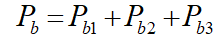2. Effective coefficient of discharge: Kd =0.65 (if Kd is not entered by user).

3. Determine correction factor for back pressure:

1. For conventional and Pilot operated valves, Kw =1.

2. For Bellows valve:

 Case Pb / Ps <0.16: Kw =1 Case 0.16 < Pb / Ps < 0.5: Kw = 1.152 -- 0.95•Pb / Ps Case Pb / Ps >0.5: Kw = 0.677
4. Calculate upstream relieving pressure:

*Pup[psi-g]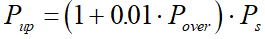5. Estimate required effective discharge area:

*W[US gal/min], Pup [psi-g]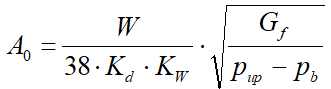6. Calculate Reynolds number: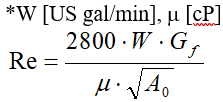7. Calculate correction factor for viscosity: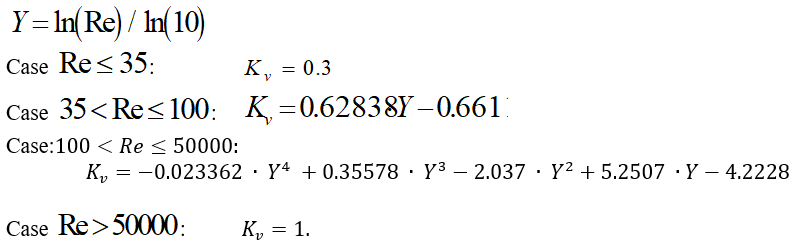8. Calculate required effective discharge area:

1. With Rupture disk: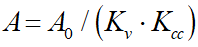2. Without Rupture disk: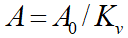Not requiring ASME capacity certification

1. Calculate total back pressure: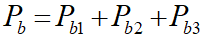2. Effective coefficient of discharge: Kd =0.62 (if Kd is not entered by user).

3. Determine correction factor for back pressure:

1. For conventional and Pilot operated valves, Kw =1.

2. For Bellows valve:

 Case Pb / Ps <0.16: Kw =1 Case 0.16 < Pb / Ps < 0.5: Kw = 1.152 -- 0.95•Pb / Ps Case Pb / Ps >0.5: Kw = 0.677
4. Calculate correction factor for overpressure: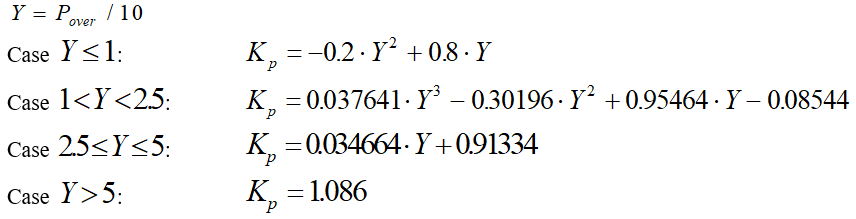5. Estimate required effective discharge area:

*W[US gal/min]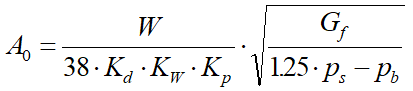6. Calculate Reynolds number: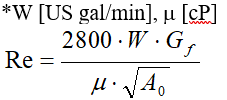7. Calculate correction factor for viscosity: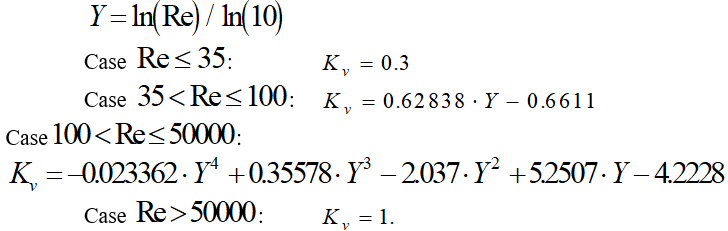8. Calculate required effective discharge area:

1. With Rupture disk:2. Without Rupture disk: